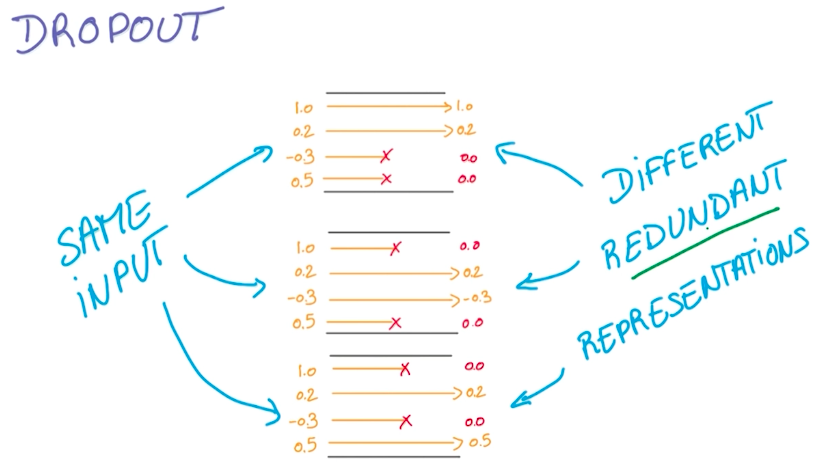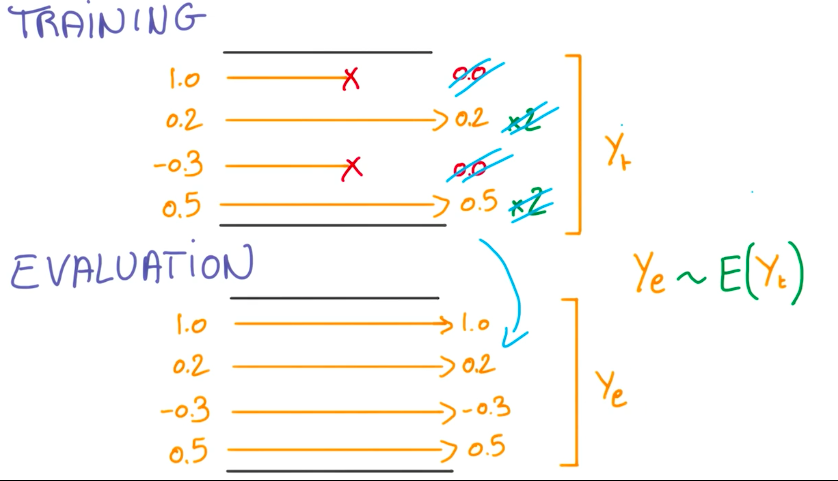# 深度学习笔记 (二) 在TensorFlow上训练一个多层卷积神经网络

## 下载并导入mnist数据集

import input_data
mnist = input_data.read_data_sets('MNIST_data', one_hot=True)

## 进入一个交互式的TensorFlow会话

TensorFlow实际上对应的是一个C++后端，TensorFlow使用会话(Session)与后端连接。通常，我们都会先创建一个图，然后再在会话(Session)中启动它。而InteractiveSession给了我们一个交互式会话的机会，使得我们可以在运行图(Graph)的时候再插入计算图，否则就要在启动会话之前构建整个计算图。使用InteractiveSession会使得我们的工作更加便利，所以大部分情况下，尤其是在交互环境下，我们都会选择InteractiveSession。

import tensorflow as tf
sess = tf.InteractiveSession()

## 利用占位符处理输入数据

x = tf.placeholder("float", shape=[None,784])

x代表的是输入图片的浮点数张量，因此定义dtype为"float"。其中，shape的None代表了没有指定张量的shape，可以feed任何shape的张量，在这里指batch的大小未定。一张mnist图像的大小是2828，784是一张展平的mnist图像的维度，即2828＝784。

y_ = tf.placeholder("float", shape=[None,10])

## 权重与偏置项初始化

ReLU对应的图像是下图左边的函数图像。#初始化权重
def weight_variable(shape):
initial = tf.truncated_normal(shape, stddev = 0.1)
return tf.Variable(initial)
#初始化偏置项
def bias_variable(shape):
initial = tf.constant(0.1, shape = shape )
return tf.Variable(initial)

## 卷积与池化

#卷积过程
def conv2d(x,w):
return tf.nn.conv2d(x,w,
strides=[1,1,1,1],padding='SAME')
#池化过程
def max_pool_2x2(x):
return tf.nn.max_pool(x,ksize=[1,2,2,1],
strides=[1,2,2,1],padding='SAME')

## 第一层卷积

(1 #28x28->32 #28x28)

w_conv1 = weight_variable([5,5,1,32])
b_conv1=bias_variable()

x_image=tf.reshape(x,[-1,28,28,1])

h_conv1=tf.nn.relu(conv2d(x_image,w_conv1)+b_conv1)

## 第一次池化

(32 #28x28->32 #14x14)

h_pool1=max_pool_2x2(h_conv1)

## 第二层卷积与第二次池化

(32 #14x14->64 #14x14->64 #7x7)

w_conv2=weight_variable([5,5,32,64])
b_conv2=bias_variable()

h_conv2=tf.nn.relu(conv2d(h_pool1,w_conv2)+b_conv2)
h_pool2=max_pool_2x2(h_conv2)

## 密集连接层

w_fc1=weight_variable([7*7*64,1024])
b_fc1=bias_variable()

h_pool2_flat=tf.reshape(h_pool2,[-1,7*7*64])
h_fc1=tf.nn.relu(tf.matmul(h_pool2_flat,w_fc1)+b_fc1)

## Dropout

keep_prob=tf.placeholder("float")
h_fc1_drop=tf.nn.dropout(h_fc1,keep_prob)## 类别预测与输出

w_fc2=weight_variable([1024,10])
b_fc2=bias_variable()

y_conv=tf.nn.softmax(tf.matmul(h_fc1_drop,w_fc2)+b_fc2)

## 模型的评价

#计算交叉熵的代价函数
cross_entropy = -tf.reduce_sum(y_*tf.log(y_conv))
#使用优化算法使得代价函数最小化
train_step = tf.train.AdamOptimizer(1e-4).minimize(cross_entropy)
#找出预测正确的标签
correct_prediction = tf.equal(tf.argmax(y_conv,1), tf.argmax(y_,1))
#得出通过正确个数除以总数得出准确率
accuracy = tf.reduce_mean(tf.cast(correct_prediction, "float"))
sess.run(tf.initialize_all_variables())
#每100次迭代输出一次日志，共迭代20000次
for i in range(20000):
batch = mnist.train.next_batch(50)
if i%100 == 0:
train_accuracy = accuracy.eval(feed_dict={
x:batch, y_: batch, keep_prob: 1.0})
print "step %d, training accuracy %g"%(i, train_accuracy)
train_step.run(feed_dict={x: batch, y_: batch, keep_prob: 0.5})

print "test accuracy %g"%accuracy.eval(feed_dict={
x: mnist.test.images, y_: mnist.test.labels, keep_prob: 1.0})

TensorFlow官方文档

Udacity-Deep Learning